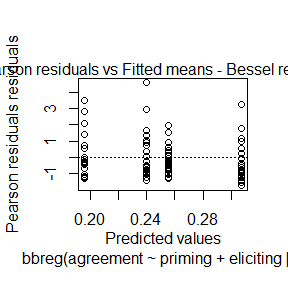# Introduction to bbreg

library(bbreg)

# Brief introduction

The bbreg package deals with regression models with response variables being continuous and bounded. It currently provides implementation of two regression models: beta regression https://www.tandfonline.com/doi/abs/10.1080/0266476042000214501 and bessel regression https://arxiv.org/abs/2003.05157. For both of these models, the estimation is done with the EM-algorithm. The EM-algorithm approach for beta regression was developed in https://www.tandfonline.com/doi/abs/10.1080/00949655.2017.1350679.

# Choosing between bessel regression and beta regression

There are several manners to choose between bessel and beta regression, most of them using diagnostic analysis. One approach we would like to call attention is the discrimination test developed in the bessel regression paper https://arxiv.org/abs/2003.05157. This discrimination test has a very nice performance on selecting the “correct” distribution (in the sense that it performs well with synthetic data) and is implemented in the bbreg package.

Another approach to select between bessel and beta regression models is to use quantile-quantile plots with simulated envelopes. The bbreg package also has a function to build such plots.

We will show below how to use the discrimination test and how to construct the quantile-quantile plot with simulated envelopes.

# Using the bbreg package

From version 2.0.0 onward, the usage of the bbreg package is standard in the sense that it uses formula to handle data frames and that it has several standard S3 methods implemented, such as summary, plot, fitted, predict, etc.

If the regression model is not specified (via the model parameter), the discrimination test is used to select the regression model to be used.

## Fitting bbreg (bessel or beta regression) models

Let us fit a model:

fit <- bbreg(agreement ~ priming + eliciting, data = WT)

Let us now see its output (notice that it provides a nice print when the fitted object is called):

fit
#>
#> Bessel regression via EM - Model selected via Discrimination test (DBB)
#>
#> Call:
#> bbreg(agreement ~ priming + eliciting | 1)
#>
#> Coefficients modeling the mean (with logit link):
#> (intercept)     priming   eliciting
#>  -1.1537851  -0.2548633   0.3392742
#> Coefficients modeling the precision (with identity link):
#> (intercept)
#>    4.924825

It is also noteworthy that the bessel regression model was chosen based on the discrimination criteria. If we want to fit a beta regression model (with estimates obtained from the EM algorithm, which are more robust than the usual maximum likelihood estimates due to the flatness of the likelihood function with respect to the precision parameter), we pass the argument model = “beta”:

fit_beta <- bbreg(agreement ~ priming + eliciting, data = WT, model = "beta")
fit_beta
#>
#> Beta regression via EM - Ignoring the Discrimination test (DBB)
#>
#> Call:
#> bbreg(agreement ~ priming + eliciting | 1)
#>
#> Coefficients modeling the mean (with logit link):
#> (intercept)     priming   eliciting
#>  -1.1354350  -0.3003169   0.3307395
#> Coefficients modeling the precision (with identity link):
#> (intercept)
#>    7.657369

Observe that the bbreg package makes it explicit that the discrimination test was ignored and the beta regression was perfomed.

Let us now add a precision covariate. Let us add the covariate priming as precision covariate. To this end, we add “| priming” to the end of the formula:

fit_priming <- bbreg(agreement ~ priming + eliciting | priming, data = WT)
fit_priming
#>
#> Bessel regression via EM - Model selected via Discrimination test (DBB)
#>
#> Call:
#> bbreg(agreement ~ priming + eliciting | priming)
#>
#> Coefficients modeling the mean (with logit link):
#> (intercept)     priming   eliciting
#>  -1.1192967  -0.4062379   0.3763049
#> Coefficients modeling the precision (with log link):
#> (intercept)     priming
#>   1.3195882   0.7192713

To add both priming and eliciting as precision covariates, we add “|priming + eliciting” to the end of the formula:

fit_priming_eliciting <- bbreg(agreement ~ priming + eliciting | priming + eliciting, data = WT)
fit_priming_eliciting
#>
#> Bessel regression via EM - Model selected via Discrimination test (DBB)
#>
#> Call:
#> bbreg(agreement ~ priming + eliciting | priming + eliciting)
#>
#> Coefficients modeling the mean (with logit link):
#> (intercept)     priming   eliciting
#>  -1.0987423  -0.3954700   0.3318582
#> Coefficients modeling the precision (with log link):
#> (intercept)     priming   eliciting
#>   1.2100896   0.7159740   0.2146362

## Viewing summaries

To view a summary of the fitted model, we simply call the method summary to the fitted bbreg object:

summary(fit)
#>
#> Bessel regression via EM - Model selected via Discrimination test (DBB):
#> Call:
#> bbreg(agreement ~ priming + eliciting | 1)
#> Number of iterations of the EM algorithm = 297
#>
#>  Results of the discrimination test DBB:
#>     sum(z2/n) sum(quasi_mu)    |D_bessel|      |D_beta|
#>        0.0853       52.6201        0.0004        0.0030
#>
#>  Pearson residuals:
#>      RSS      Min       1Q   Median       3Q      Max
#> 331.9432  -1.7387  -0.6606  -0.3847   0.5562   4.5786
#>
#>  Coefficients modeling the mean (with logit link):
#>             Estimate Std.error z-value Pr(>|z|)
#> (intercept) -1.15379   0.05251 -21.971  < 2e-16 ***
#> priming     -0.25486   0.05733  -4.446 8.77e-06 ***
#> eliciting    0.33927   0.05846   5.804 6.49e-09 ***
#>
#>  Coefficients modeling the precision (with identity link):
#>             Estimate Std.error z-value Pr(>|z|)
#> (intercept)   4.9248    0.4493   10.96   <2e-16 ***
#> g(phi) = 0.1316
#> ---
#>  Signif. codes:  0 '***' 0.001 '**' 0.01 '*' 0.05 '.' 0.1 ' ' 1

For the beta regression fit:

summary(fit_beta)
#>
#> Beta regression via EM - Ignoring the Discrimination test (DBB):
#> Call:
#> bbreg(agreement ~ priming + eliciting | 1)
#> Number of iterations of the EM algorithm = 173
#>
#>  Pearson residuals:
#>      RSS      Min       1Q   Median       3Q      Max
#> 377.2069  -1.8658  -0.6997  -0.3824   0.5793   4.8412
#>
#>  Coefficients modeling the mean (with logit link):
#>             Estimate Std.error z-value Pr(>|z|)
#> (intercept) -1.13543   0.07076 -16.046  < 2e-16 ***
#> priming     -0.30032   0.08101  -3.707  0.00021 ***
#> eliciting    0.33074   0.08083   4.092 4.28e-05 ***
#>
#>  Coefficients modeling the precision (with identity link):
#>             Estimate Std.error z-value Pr(>|z|)
#> (intercept)   7.6574    0.5632    13.6   <2e-16 ***
#> g(phi) = 0.1155
#> ---
#>  Signif. codes:  0 '***' 0.001 '**' 0.01 '*' 0.05 '.' 0.1 ' ' 1

For the fit with priming as covariates:

summary(fit_priming)
#>
#> Bessel regression via EM - Model selected via Discrimination test (DBB):
#> Call:
#> bbreg(agreement ~ priming + eliciting | priming)
#> Number of iterations of the EM algorithm = 221
#>
#>  Results of the discrimination test DBB:
#>     sum(z2/n) sum(quasi_mu)    |D_bessel|      |D_beta|
#>        0.0853       52.6201        0.0002        0.0026
#>
#>  Pearson residuals:
#>      RSS      Min       1Q   Median       3Q      Max
#> 341.2741  -1.6532  -0.6773  -0.3175   0.6081   4.3879
#>
#>  Coefficients modeling the mean (with logit link):
#>             Estimate Std.error z-value Pr(>|z|)
#> (intercept) -1.11930   0.05584 -20.043  < 2e-16 ***
#> priming     -0.40624   0.06034  -6.733 1.67e-11 ***
#> eliciting    0.37630   0.05694   6.609 3.87e-11 ***
#>
#>  Coefficients modeling the precision (with log link):
#>             Estimate Std.error z-value Pr(>|z|)
#> (intercept)   1.3196    0.1304  10.117  < 2e-16 ***
#> priming       0.7193    0.1816   3.961 7.46e-05 ***
#> ---
#>  Signif. codes:  0 '***' 0.001 '**' 0.01 '*' 0.05 '.' 0.1 ' ' 1

## Getting fitted values

If we want to get the fitted values, we can use the fitted method on the fitted bbreg object. We can have four types of fitted values: the response, which provides the fitted means, the link which provides the the fitted linear predictors for the means, the precision which provides the fitted precisions and variance which provides the fitted variances for the response variables.

For example, consider the fit object in the first example. We can obtain the fitted means by simply calling the fitted method:

fitted_means <- fitted(fit)
#>         1         2         3         4         5         6
#> 0.2397984 0.2397984 0.2397984 0.2397984 0.2397984 0.2397984

and by passing the argument type = “variance”, we get:

fitted_variances <- fitted(fit, type = "variance")
#>          1          2          3          4          5          6
#> 0.02399282 0.02399282 0.02399282 0.02399282 0.02399282 0.02399282

## Making predictions

To get predictions, we use the predict method on the fitted bbreg object. We can pass an additional argument newdata equal to a data frame containing the values of the covariates to get predictions based on these covariates. If one does not pass the newdata argument, this functions returns the fitted values (that is, if the newdata parameter is NULL, then this method is identical to the fitted method).

Let us create a data frame containing new covariates for the fitted model fit_priming_eliciting:

new_data_example <- data.frame(priming = c(0,0,1), eliciting = c(0,1,1))

Now, let us obtain the corresponding predicted mean values and predicted variances for the response variables:

predict(fit_priming_eliciting, newdata = new_data_example)
#>         1         2         3
#> 0.2499756 0.3171535 0.2382398
predict(fit_priming_eliciting, newdata = new_data_example, type = "variance")
#>          1          2          3
#> 0.03150342 0.03186165 0.01609758

It is interesting to observe that the above fitted model has priming and eliciting as covariates for both the mean and the precision parameters. We do not have to worry about that, the bbreg handles it automatically.

The same new_data_example can also be used for the fitted model fit, which does not have precision covariates (priming and eliciting are covariates for the mean):

predict(fit, newdata = new_data_example)
#>         1         2         3
#> 0.2397984 0.3069301 0.2555221
predict(fit, newdata = new_data_example, type = "variance")
#>          1          2          3
#> 0.02399282 0.02799772 0.02503724

In short, the data frame to be passed as newdata must contain all the mean and precision covariates as columns, the bbreg package handles the rest.

Lastly, observe that if we do not pass the argument newdata, the predict method coincides with the fitted method:

predict_without_newdata <- predict(fit)
identical(predict_without_newdata, fitted_means)
#>  TRUE

## Creating simulated envelopes

To create simulated envelopes for a bbreg object, we just need to set the number of random draws to be made at the envelope argument of the bbreg function:

fit_envelope <- bbreg(agreement ~ priming + eliciting, envelope = 300, data = WT)

Observe that the bbreg function (through the usage of the pbapply package) provides a nice progress bar with an estimate of the remaining time to complete the simulation.

The summary method provides the percentage of data within the bands formed by the simulated envelopes. This can also be used as a criterion to select between the bessel and beta regressions.

summary(fit_envelope)
#>
#> Bessel regression via EM - Model selected via Discrimination test (DBB):
#> Call:
#> bbreg(agreement ~ priming + eliciting | 1)
#> Number of iterations of the EM algorithm = 297
#>
#>  Results of the discrimination test DBB:
#>     sum(z2/n) sum(quasi_mu)    |D_bessel|      |D_beta|
#>        0.0853       52.6201        0.0004        0.0030
#>
#>  Pearson residuals:
#>      RSS      Min       1Q   Median       3Q      Max
#> 331.9432  -1.7387  -0.6606  -0.3847   0.5562   4.5786
#>
#>  Coefficients modeling the mean (with logit link):
#>             Estimate Std.error z-value Pr(>|z|)
#> (intercept) -1.15379   0.05251 -21.971  < 2e-16 ***
#> priming     -0.25486   0.05733  -4.446 8.77e-06 ***
#> eliciting    0.33927   0.05846   5.804 6.49e-09 ***
#>
#>  Coefficients modeling the precision (with identity link):
#>             Estimate Std.error z-value Pr(>|z|)
#> (intercept)   4.9248    0.4493   10.96   <2e-16 ***
#> g(phi) = 0.1316
#> ---
#>  Signif. codes:  0 '***' 0.001 '**' 0.01 '*' 0.05 '.' 0.1 ' ' 1
#> Percentage of residual within the envelope = 33.6232

The fitted bbreg object with simulated envelopes can be used to produce a quantile-quantile plot with simulated envelopes.

## Diagnostic plots for bbreg objects

The bbreg package comes with a plot method that currently has four kinds of plots implemented: Residuals vs. Index; Q-Q Plot (if the fit contains simulated envelopes, the plot will be with the simulated envelopes); Fitted means vs. Response and Residuals vs. Fitted means.

To produce all four plots, one may simply apply the plot method to the bbreg object:

plot(fit)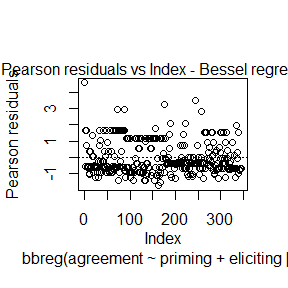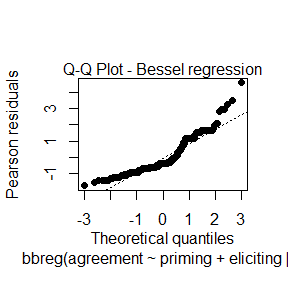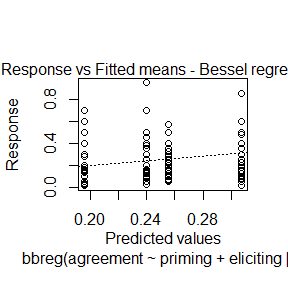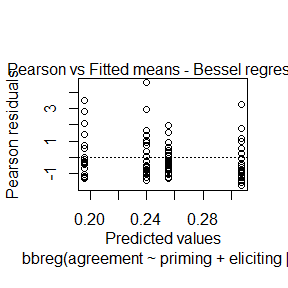Notice that since there were no simulated envelopes for the fit object, the Q-Q plot does not contain simulated envelopes. Nevertheless, it contains a line connecting the first and third quartiles of the normal distribution (one can remove this line by setting the argument qqline to FALSE).

To choose a specific plot, one can set the parameter which to a vector containing the numbers of the plots to be shown. The plot numbers are given by: Plot 1: Residuals vs. Index; Plot 2: Q-Q Plot (if the fit contains simulated envelopes, the plot will be with the simulated envelopes); Plot 3: Fitted means vs. Response; Plot 4: Residuals vs. Fitted means.

For instance, if we only want to plot the Q-Q plot, we set the argument which to 2:

plot(fit_envelope, which = 2)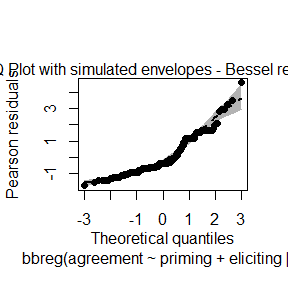Notice that since the fit_envelope object contains simulated envelopes, the Q-Q plot is displayed with simulated envelopes.

Finally, to avoid being asked between plots, just set the ask parameter to FALSE:

plot(fit, which = c(1,4), ask = FALSE)